# MCAT Physical : Acid-Base Equilibrium

## Example Questions

← Previous 1

### Example Question #27 : Mcat Physical Sciences

Acids and bases can be described in three principal ways. The Arrhenius definition is the most restrictive. It limits acids and bases to species that donate protons and hydroxide ions in solution, respectively. Examples of such acids include HCl and HBr, while KOH and NaOH are examples of bases. When in aqueous solution, these acids proceed to an equilibrium state through a dissociation reaction.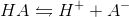All of the bases proceed in a similar fashion.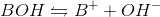The Brønsted-Lowry definition of an acid is a more inclusive approach. All Arrhenius acids and bases are also Brønsted-Lowry acids and bases, but the converse is not true. Brønsted-Lowry acids still reach equilibrium through the same dissociation reaction as Arrhenius acids, but the acid character is defined by different parameters. The Brønsted-Lowry definition considers bases to be hydroxide donors, like the Arrhenius definition, but also includes conjugate bases such as the A- in the above reaction. In the reverse reaction, A- accepts the proton to regenerate HA. The Brønsted-Lowry definition thus defines bases as proton acceptors, and acids as proton donors.

Which of the following expressions most closely approximates the equilibrium constant of pure water?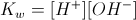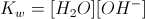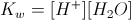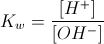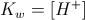Explanation:

The autionization of water proceeds as follows: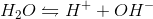The equilibrium constant is calculated by the following formula.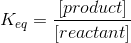The equilibrium expression is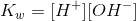, with the pure water reactant excluded. Pure solids and liquids are not included in the equilibrium calculation.

### Example Question #28 : Mcat Physical Sciences

What is the hydroxide concentration in an aqueous solution with a pH of 2?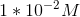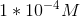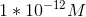There are no hydroxide ions in the solutionExplanation:

The hydroxide concentration can be determined by considering the autoionization of water in a solution. At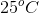, water has the equilibrium constant of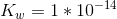. This value is based on the autoionization of water.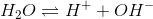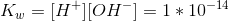Since the reaction is in terms of proton and hydroxide ion concentrations, we can set the expression equal to this value in order to determine the concentration of hydroxide ions at the given pH. We start by determining the concentration of protons in the solution by using the following equation: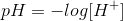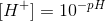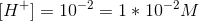Now, we can solve for the hydroxide concentration.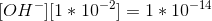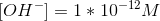This problem can also be solved by calculating the pOH of the solution and using this value to find the hydroxide ion concentration.

### Example Question #29 : Mcat Physical Sciences

What is the pH of a solution which has a hydroxide ion concentration of 5 * 10-4M?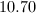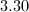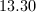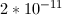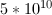Explanation:

First convert concentration of OH- into concentration of H+. Remember that Kw is 1*10-14.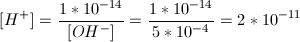Then, convert concentration of H+ into pH.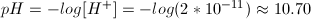Alternatively, you can use the hydroxide concentration to solve for pOH and convert to pH.

### Example Question #1 : Acid Base Equilibrium

Diffusion can be defined as the net transfer of molecules down a gradient of differing concentrations. This is a passive and spontaneous process and relies on the random movement of molecules and Brownian motion. Diffusion is an important biological process, especially in the respiratory system where oxygen diffuses from alveoli, the basic unit of lung mechanics, to red blood cells in the capillaries.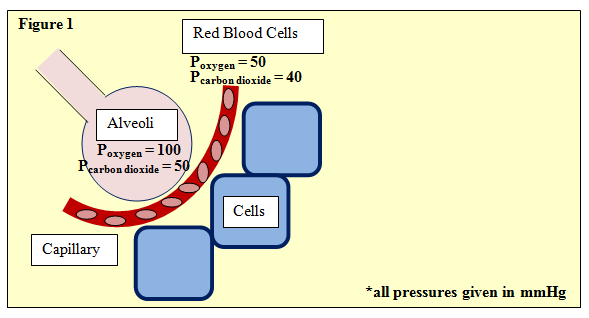Figure 1 depicts this process, showing an alveoli separated from neighboring cells by a capillary with red blood cells. The partial pressures of oxygen and carbon dioxide are given. One such equation used in determining gas exchange is Fick's law, given by:

ΔV = (Area/Thickness) · Dgas · (P1 – P2)

Where ΔV is flow rate and area and thickness refer to the permeable membrane through which the gas passes, in this case, the wall of the avlveoli. P1 and P2 refer to the partial pressures upstream and downstream, respectively. Further, Dgas­, the diffusion constant of the gas, is defined as:

Dgas = Solubility / (Molecular Weight)^(1/2)

Which following pair gives the correct equation, and change in pH, when carbon dioxide diffuses into blood?

CO2 + H2O   H2CO3 | pH increases

O2 + CO2 + H2O  H2O2 + CO32– | pH decreases

CO2 + H2O   HCO3– | pH decreases

CO2 + H2O   H2CO3 | pH decreases

CO2 + H2O   H2CO3 | pH decreases

Explanation:

This is essentially a two-part question. The first asks you to generate the production of carbonic acid; and the second, requires you to infer that an acid will lower blood pH. Only one answer choice is both balanced correctly and gives an accurate change in pH. Although this knowledge is not required for the MCAT, your body constantly monitors blood pH to determine if concentrations of CO2 ­are too high, or alternatively, if O2 concentrations are too low.

### Example Question #1 : Reactions And Titrations

As the value of Ka increases, __________

the reaction HA → H+ + A favors the products

pKa decreases

the strength of the conjugate base decreases

the strength of the acid increases

all of these are true

all of these are true

Explanation:

Ka represents the equilibrium constant for the acid dissociation in water, HA → H+ A, so it is a measure of the products divided by the reactants. As this value increases, the reaction favors the products (the ions) more, meaning that the acid dissociates more. The definition of a strong acid is one that fully dissociates in water, so as Ka increases the strength of the acid increases, and the strength of the conjugate base decreases. pKa is defined as the –log(Ka), so as Ka increases pKa decreases. All of these answer choices are correct.

### Example Question #2 : Reactions And Titrations

What is the pKa of acetic acid? (K= 1.8 * 10–5)

8.6

4.7

4.2

5.3

2.1

4.7

Explanation:

We know that pKa is equal to –log(Ka). Thus, pKa of acetic acid is –log(1.8 * 10–5). This is not an easy problem to solve in your head, but there is a trick.

We know that 1 * 10–4 > 1.8 * 10–5 > 1 * 10–5, and we know that –log(1 * 10–4) = 4 and –log(1 * 10–5) = 5. Now we can conclude that our pKa is somewhere between 4 and 5.

Two answer choices fall in this range: 4.2 and 4.7.

1.8 * 10–5 is closer to 1 * 10–5 than it is to 1 * 10–4, so we can pick the answer closer to 5 than to 4 : 4.7.

### Example Question #3 : Reactions And Titrations

HCN dissociates based on the following reaction.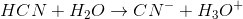The Ka for hydrogen cyanide is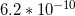.

What is the Kfor CN-?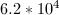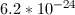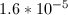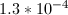Explanation:

Remember that the Ka for the acid and the Kb for the conjugate base, when multiplied will equal the autoionization of water constant (Kw).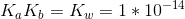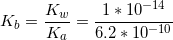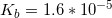### Example Question #1 : Acid Base Equilibrium

 Compound Base strength, Kb 1 1012 2 105 3 101 4 10-8

Students in a chemistry class are given one of four unknown samples in a laboratory. A student is told that his compound is the strongest acid of the four compounds. Based on the information in the above table, which compound was the student given?

Compound 3

Compound 2

Compound 1

Compound 4

Compound 4

Explanation:

The strongest acid of the group will also have the smallest Kb value. As the weakest base (smallest Kb), compound 4 will only partially dissociate in solution because it has a fairly strong conjugate acid. Were this question asking which base was the strongest, compound 1 would be the answer, due to its large Kb value.

### Example Question #5 : Reactions And Titrations

Acids and bases can be described in three principal ways. The Arrhenius definition is the most restrictive. It limits acids and bases to species that donate protons and hydroxide ions in solution, respectively. Examples of such acids include HCl and HBr, while KOH and NaOH are examples of bases. When in aqueous solution, these acids proceed to an equilibrium state through a dissociation reaction.All of the bases proceed in a similar fashion.The Brønsted-Lowry definition of an acid is a more inclusive approach. All Arrhenius acids and bases are also Brønsted-Lowry acids and bases, but the converse is not true. Brønsted-Lowry acids still reach equilibrium through the same dissociation reaction as Arrhenius acids, but the acid character is defined by different parameters. The Brønsted-Lowry definition considers bases to be hydroxide donors, like the Arrhenius definition, but also includes conjugate bases such as the A- in the above reaction. In the reverse reaction, A- accepts the proton to regenerate HA. The Brønsted-Lowry definition thus defines bases as proton acceptors, and acids as proton donors.

In aqueous conditions the equilibrium constant for a Brønsted-Lowry base,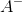, can be expressed as which of the following?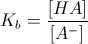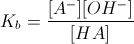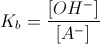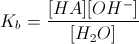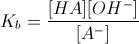Explanation:

The base reaction will essentially be the reverse of the acid reaction. In the question, the aqueous conditions mean that the base,, reacts with water to give the following reaction: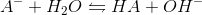Following normal equilibrium convention, we omit water from the equation because it is a pure liquid.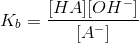### Example Question #6 : Reactions And Titrations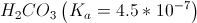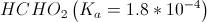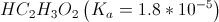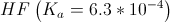Given the above values of Ka, place the acids in order from strongest to weakest.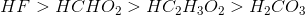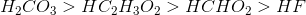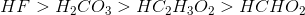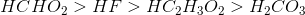None of the above.